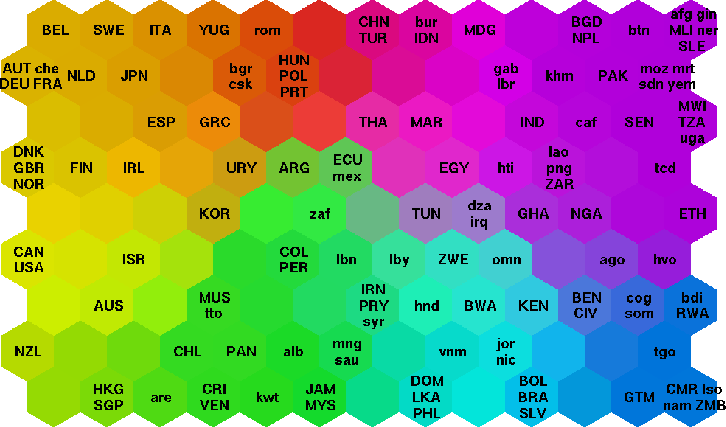# a quick note on visualizing topic models as self organizing map

I wanted to visualize topic models as a self-organizing map. This code snippet was helpful. (Here’s its blog post).

In my standard topic modeling script in R, I added this:

```library("kohonen")
doc.topics.sc <- scale(doc.topics)
set.seed(80)
doc.topics.som <- som(doc.topics.sc, grid = somgrid(20, 16, "hexagonal"))
plot(doc.topics.som, main = "Self Organizing Map of Topics in Documents")
```

which gives something like this:Things to be desired: I don’t know which circle represents what document. Each pie slice represents a topic. If you have more than around 10 topics, you get a graph in the circle instead of a pie slice. I was colouring in areas by main pie slice colour in inkscape, but then the whole thing crashed on me. Still, a move in the right direction for getting a sense of the landscape of your entire corpus. What I’m eventually hoping for is to end up with something like this (from this page):update

I found this: https://github.com/geoss/som_visualization_r which seems to work. In my topic model script, I need to save the doc.topics output as Rdata:

```save(doc.topics, file = "doctopics.RData")
```

and then the following:

```library(kohonen)

##Code for Plots
source("somComponentPlanePlottingFunction.R")
### source("Map_COUNTY_BMU.R") <- not necessary for SG
source("plotUMatrix.R")

## data is from a topic model of student writing in Eric's class

#Build SOM
aGrid <- somgrid(xdim = 20, ydim = 16, topo="hexagonal")

##NEXT LINE IS SLOW!!!
##Rlen is arbitrarily low
aSom <- som(data=as.matrix(scale(doc.topics)), grid=aGrid, rlen=1, alpha=c(0.05, 0.01), keep.data=FALSE)

##VISUALIZE RESULTS
##COMPONENT PLANES
dev.off()
par(mar = rep(1, 4))
cplanelay <- layout(matrix(1:8, nrow=4))
vars <- colnames(aSom\$data)
for(p in vars) {
plotCplane(som_obj=aSom, variable=p, legend=FALSE, type="Quantile")
}
plot(0, 0, type = "n", axes = FALSE, xlim=c(0, 1),
ylim=c(0, 1), xlab="", ylab= "")
par(mar = c(0, 0, 0, 6))
image.plot(legend.only=TRUE, col=rev(designer.colors(n=10, col=brewer.pal(9, "Spectral"))), zlim=c(-1.5,1.5))
##END PLOT

##PLOT U-MATRIX
dev.off()
plotUmat(aSom)

plot(aSom)
```

…does the trick. Notice ‘doc.topics’ makes another appearance there – I’ve got the topic model loaded into memory. Also in ‘aGrid’ the x and y have to multiply to the max number of observations you’ve got. Not enough: no problem. More than what you’ve got: you’ll get error messages. So, here’s what I ended up with:Now I just need to figure out how to put labels on each hexagonal bin. By the way, the helper functions have to be in your working directory for ‘source’ to find them.